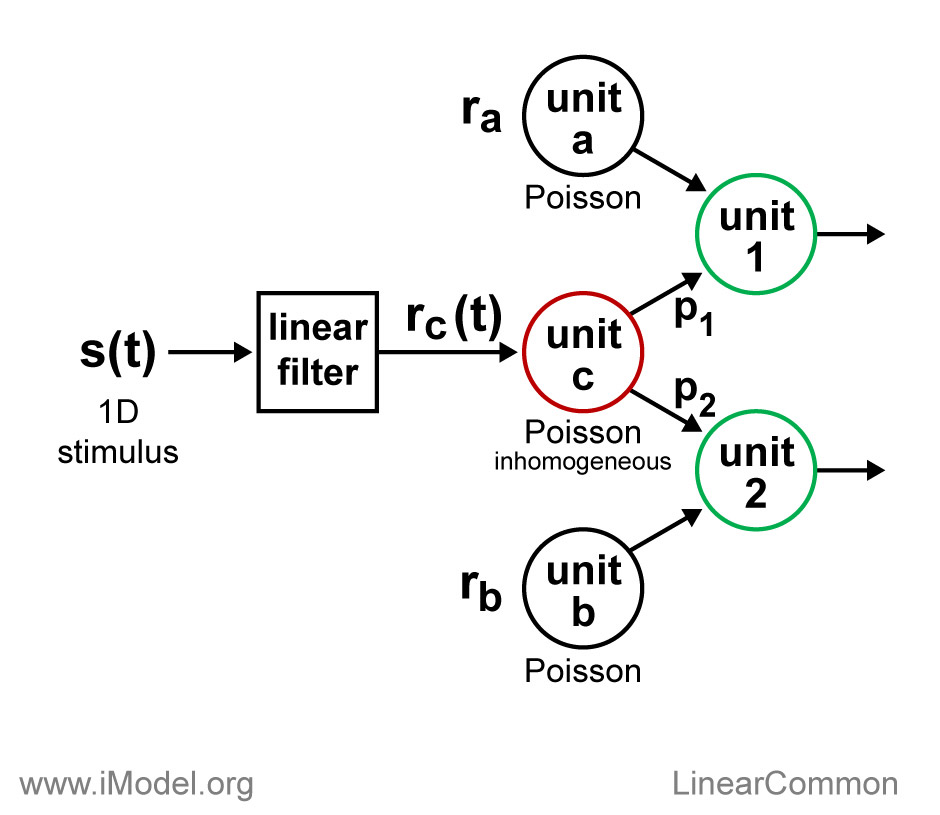iModel
Related models

LinearCommon

Variations

LinearCommon
Linear filter, Common input, Poisson
Summary

This 1D linear filter model generates a stimulus-modulated source of Poisson common input that drives units in a second stage. The second stage units simply combine the common input spikes with spikes from independent Poisson sources. This model was designed for testing spike train cross-correlation analyses and for educational exercises.The stimulus, s(t), is convolved with a linear filter. The output is scaled and half-wave rectified (not shown) to form a time varying rate, rc(t), that drives an inhomogeneous Poisson process. The resulting spike train, the output of unit c, is the source of common input to unit 1 and unit 2. These two second layer units accept each spike from the common input independently with probabilities p1 and p2, respectively. In addition to the common input spikes, the output spike trains of units 1 and 2 include a set of independent (homogeneous) Poisson spikes generated with rates ra and rb, respectively.

Additional parameters allow for a refractory period to be imposed on the spikes of unit c, and for the spikes of units 1 and 2 to be converted to bursts. An arbitrary number of second layer units can be defined. For detailed parameter descriptions, follow the link for the model class below.

Model Class: lin_01